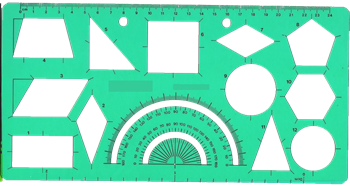# Reusing JavaScript Template Literals

Monday, March 21, 2016The word template in web programming makes one think of a reusable document or string you can combine with model data to produce output. Famous examples include Razor, Jade, and Handlebars. Template literals in JavaScript are not templates in this same sense, which is perhaps why they were originally referred to as quasi-literals in early specification work.

I've seen a number of developers become angry when thinking they can declare a template literal and then reuse the template with different inputs. Note: the following code doesn't work, but represents how one might think template literals should work.

```let template = `\${x} + \${y} = \${x+y}`;

let three = template.format({x:2, y:3});
let ten = template.format({x:5, y:5});
```

The runtime will evaluate the expressions inside the template immediately.  If x and y aren't available when the template is defined, a ReferenceError brings the script to a halt.

The easy solution, like most solutions in JavaScript, is to use a wrapper function.

```let template = (x,y) => `\${x} + \${y} = \${x+y}`;

let four = template(2,2);
let eight = template(6,2);
```

If you'd rather think in terms of model objects instead of individual parameters, add some destructuring to the parameter list.

```let template = ({x,y}) => `\${x} + \${y} = \${x+y}`;

let seven = template({x:4, y:3});
let three = template({x:1, y:2});
```

#### All My Pluralsight CoursesOdeToCode by K. Scott Allen